# Square Based Pyramid

Here we will learn about square based pyramids, including what they are and what features they have.

There are also square based pyramid worksheets based on Edexcel, AQA and OCR exam questions, along with further guidance on where to go next if you’re still stuck.

## What is a square based pyramid?

A square based pyramid is a three-dimensional shape with 4 flat triangular faces and 1 square face, 8 edges and 5 vertices.

It is sometimes called pentahedron because it has 5 faces.

A pyramid is a polyhedra with a flat base and triangular sides which meet at the apex. The flat base can be any polygon. There are different types of pyramids such as a triangular pyramid (known as a tetrahedron), a rectangular pyramid or even a hexagonal pyramid or a pentagonal pyramid.

### What is a square based pyramid?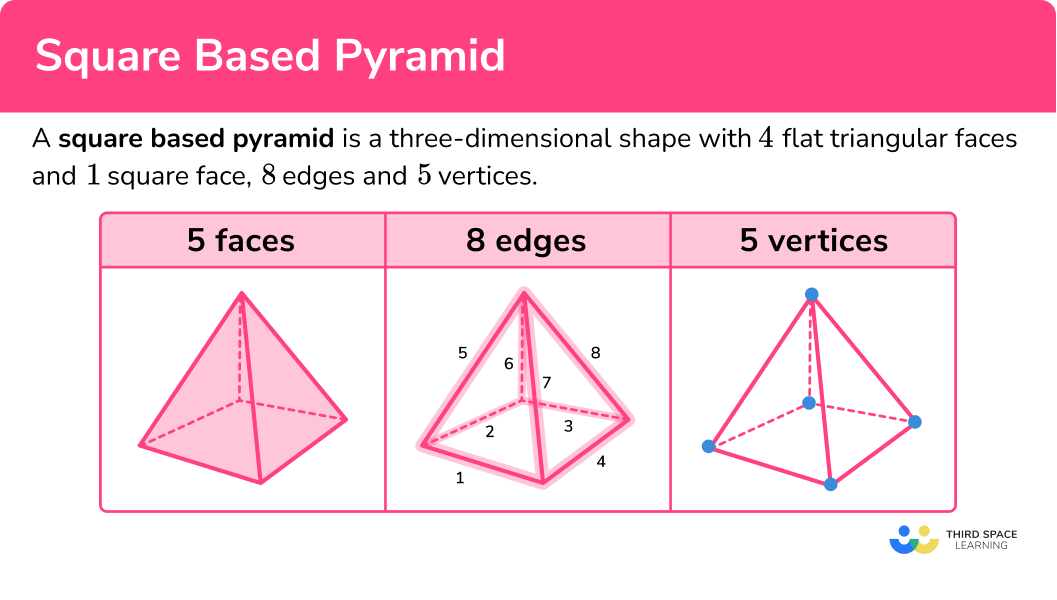### Net of a square based pyramid

The net of a square based pyramid is made of 4 triangles and a square.

### Volume of a square based pyramid

The volume of a square based pyramid can be found by using the formula

\text{Volume}=\cfrac{1}{3}\times \text{area of base} \times \text{height}.

The pyramid height should be perpendicular to its base.

This can be written as

V=\cfrac{1}{3}Bh ,

where

• V represents the volume of the pyramid,
• B represents the area of the base of the pyramid,
• h represents the perpendicular height of the pyramid.

You may not be given the perpendicular height. You may need to apply Pythagoras’ theorem to find it if you are given the slant height or the lateral edge length.

### Surface area of a square based pyramid

The surface area of a square based pyramid is calculated by finding the area of each of the faces and adding them together.

For example,

Find the surface area of the square based pyramid.

Area of a square = 5 \ cm \times 5 \ cm=25 \ cm^2

Area of a triangle = \cfrac{5 \ cm \ \times \ 8 \ cm}{2}=20 \ cm^2

There are 4 triangular faces so multiply 20 \ cm^2 by 4 to give 80 \ cm^{2}.

Total surface area = 25 \ cm^{2}+80 \ cm^{2}=105 \ cm^2

### Right square pyramid

A right square pyramid is a square based pyramid where the apex is directly above the centre of the square base, forming a right-angled triangle. The other 4 faces are isosceles triangles.

### Oblique square pyramid

An oblique square pyramid is a square based pyramid where the apex is NOT directly above the centre of the square base.

## How to calculate the volume of a square based pyramid

In order to calculate the volume of a square based pyramid:

1. Calculate the area of the base.
2. Substitute values into the formula and solve.
3. Write the answer, including the units.

### Explain how to calculate the volume of a square based pyramid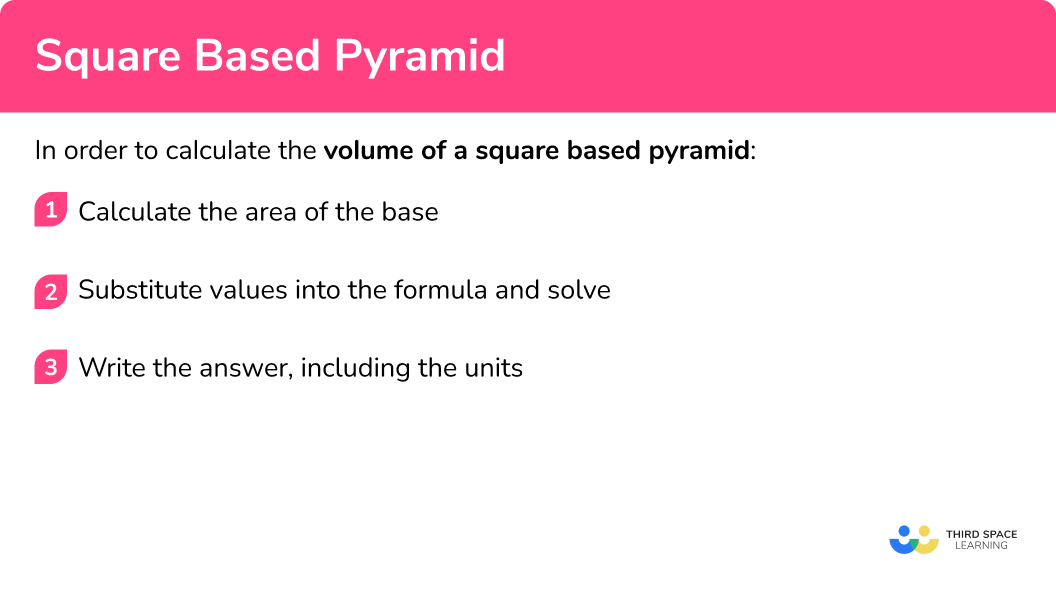## Square based pyramid examples

### Example 1: calculating the volume given the height

Find the volume of the square based pyramid.

1. Calculate the area of the base.

The area of the base can be found by squaring the base side.

7^2=49

2Substitute values into the formula and solve.

The volume of a square based pyramid can be found by using the formula,

\begin{aligned} V&=\cfrac{1}{3}Bh \\\\ V&=\cfrac{1}{3}\times 49\times 10 \\\\ V&=163.333… \end{aligned}

3Write the answer, including the units.

The volume is 163.3 \ m^3 (to 1 dp).

### Example 2: calculating the volume not given the height

Find the volume of the square based pyramid. The apex is directly above the centre of the square base.

Calculate the area of the base.

Substitute values into the formula and solve.

Write the answer, including the units.

### Example 3: calculating the height given the volume

The volume of the square based pyramid is 740 \ cm^{3}. Find the perpendicular height h.

Calculate the area of the base.

Substitute values into the formula and solve.

Write the answer, including the units.

### Example 4: calculating the length of the base given the volume

The volume of the square based pyramid is 570 \ cm^{3}. Find the base edge.

Calculate the area of the base.

Substitute values into the formula and solve.

Write the answer, including the units.

### Example 5: working out the total surface area of a square pyramid

Find the total surface area of this square based pyramid.

Calculate the area of the base.

Substitute values into the formula and solve.

Write the answer, including the units.

### Example 6: working out the total surface area using Pythagoras’ theorem

Calculate the area of the base.

Substitute values into the formula and solve.

Write the answer, including the units.

### Common misconceptions

• The height is the perpendicular height

The height of the pyramid needs to be the perpendicular height. This is the height that is at a right-angle to the base.

• Using the correct units

Remember to use cubic units for volume such as cm^3 or m^{3}. Square units are for area, for example cm^2 or m^{2}.

• Use the correct volume formula

There are many different volume formulas in maths. Make sure that you use the correct one to work out the volume of a pyramid.

\text{Volume}=\cfrac{1}{3}\times \text{area of base} \times \text{height}

Or

V=\cfrac{1}{3}Bh

• Be accurate

When there are two or more steps in your workings, do not round your workings. Only round at the end of your solution so that your answer is accurate.

• Take care with rounding

At the end of the question, make sure you round your answer to the correct number of decimal places or significant figures.

Volume of a pyramid is part of our series of lessons to support revision on pyramids. You may find it helpful to start with the main pyramid lesson for a summary of what to expect, or use the step by step guides below for further detail on individual topics. Other lessons in this series include:

### Practice square based pyramid questions

1. How many faces does a square based pyramid have?

4375A square based pyramid has 5 faces. One is the square base and the other 4 are triangles which are the lateral faces.

2. Which of these nets are for a square based pyramid?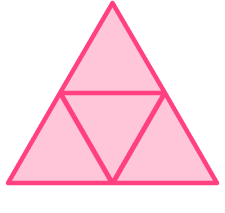A square based pyramid has 5 faces. One is a square, the base. The other faces are triangles which need to have the same slant height so that they meet together at the apex.

3. Find the volume of this square based pyramid.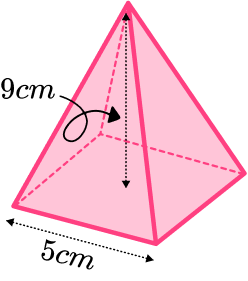405 \ cm^3135 \ cm^375 \ cm^3225 \ cm^3We can work out the volume of a pyramid by using the formula

V=\cfrac{1}{3}Bh.

The base area can be found by squaring the side length of the base square.

5^2=25

We can substitute the values we have been given into the formula and work out the volume.

V=\cfrac{1}{3}\times 25 \times 9=75

So the volume is 75 \ cm^{3}.

4. Find the volume of this square based pyramid.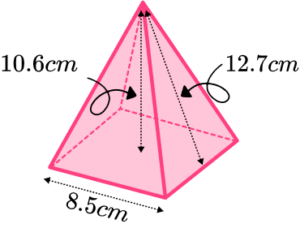255 \ cm^3305 \ cm^3256 \ cm^3306 \ cm^3We can work out the volume of a pyramid by using the formula

V=\cfrac{1}{3}Bh.

The base area can be found by squaring the side length of the base square.

8.5^2=72.25

We can substitute the values we have been given into the formula and work out the volume. We need to make sure that we use the perpendicular height.

V=\cfrac{1}{3}\times 72.25 \times 10.6=255.28…

So the volume is 255 \ cm^{3} (to 3 sf).

5. The volume of a square based pyramid is 165 \ cm^{3}. The side length of the square is 9.2. Find the perpendicular height of the square based pyramid. Give your answer correct to 3 significant figures.

5.84 \ cm7.33 \ cm5.85 \ cm7.34 \ cmWe use the formula for volume of a pyramid

V=\cfrac{1}{3}Bh.

The base area can be found by squaring the side length of the base square.

9.2^2=84.64

We can substitute the values we have been given into the formula.

165=\cfrac{1}{3}\times 84.64 \times h

We can reverse this to find the base area.

h=\cfrac{80\times 3}{9.7}=5.8482…

So the perpendicular height is 5.85 \ cm when rounded to 3 significant figures.

6. Find the total surface area of the square based pyramid. The apex is directly above the centre of the square base. Give your answer correct to 3 significant figures.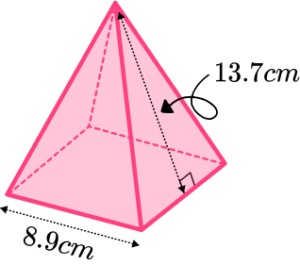323 \ cm^2432 \ cm^2140 \ cm^2253 \ cm^2A triangular based pyramid has 5 faces. The square base and 4 triangular faces. Since the apex is directly above the centre of the square base, all 4 triangles are congruent.

Area of square base,

8.9^2=79.21.

Area of triangle face,

A=\cfrac{1}{2}bh=\cfrac{1}{2}\times 8.9\times 13.7=60.965

Total surface area of square pyramid,

79.21+4\times 60.965=323.07 .

So the total surface area is 323 \ cm^2 (to 3 sf).

### Square based pyramid GCSE questions

1. Circle the correct diagram which shows the plan view of a square based pyramid.(1 mark)

D

(1)

2. (a) How many edges does a square based pyramid have?

(b) How many vertices does a square based pyramid have?

(2 marks)

(a) 8

(1)

(b) 5

(1)

3. The diagram shows a square based pyramid.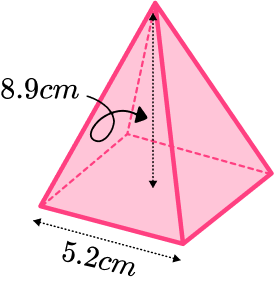Calculate the volume of this pyramid.

(4 marks)

Area of base is 5.2^{2}=27.04 .

(1)

Volume of pyramid is \cfrac{1}{3} \times 27.04 \times 8.9=80.2186…

(1)

80.2

(1)

cm^3

(1)

4. An equilateral square pyramid is a square based pyramid with equal lengths.

All the lengths are 10 \ cm.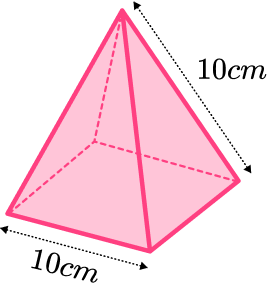Find the total surface area of the equilateral square based pyramid.

(5 marks)

Area of base is 10^{2}=100 .

(1)

Height of triangle face 5\sqrt{3}

Or sin(60)=\cfrac{\sqrt{3}}{2}

(1)

Area of one triangle is 25\sqrt{3} .

(1)

4 \times 25\sqrt{3}

(1)

100+100\sqrt{3} \ cm^2

(1)

## Learning checklist

You have now learned how to:

• Recognise a square based pyramid and its features
• Calculate the volume of a square based pyramid
• Solve problems involving the volume
• Find the total surface area of a square based pyramid

## Still stuck?

Prepare your KS4 students for maths GCSEs success with Third Space Learning. Weekly online one to one GCSE maths revision lessons delivered by expert maths tutors.

Find out more about our GCSE maths tuition programme.